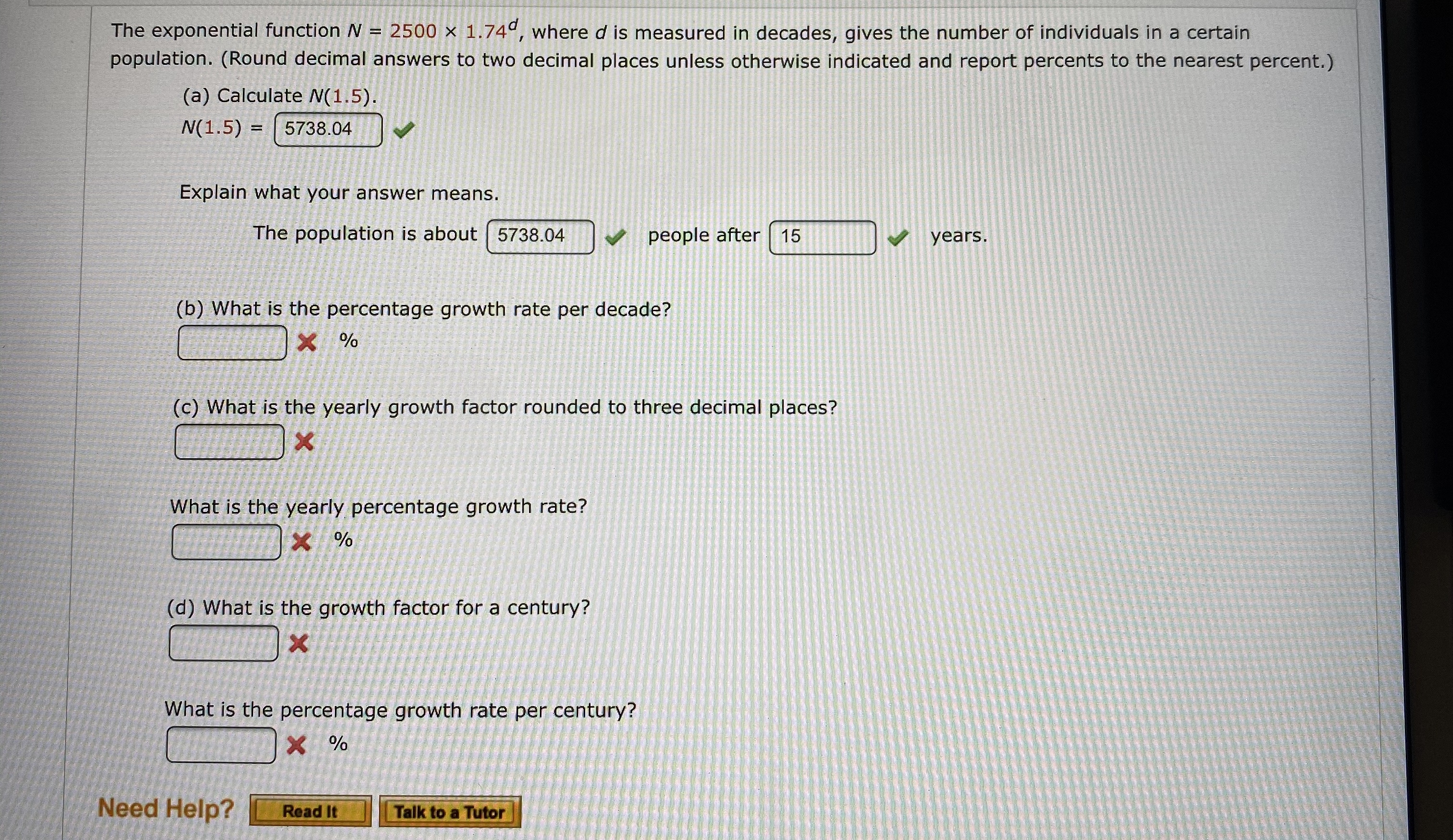# 2500 x 1.74, where d is measured in decades, gives the number of individuals in a certainThe exponential function Mpopulation. (Round decimal answers to two decimal places unless otherwise indicated and report percents to the nearest percent.)(a) Calculate N(1.5).N(1.5) =5738.04Explain what your answer means.The population is about 5738.04people after 15years.(b) What is the percentage growth rate per decade?X %(c) What is the yearly growth factor rounded to three decimal places?XWhat is the yearly percentage growth rate?X%(d) What is the growth factor for a century?XWhat is the percentage growth rate per century?XNeed Help?Read ItTalk to a Tutor

Question
10 views

What are the blanks?help_outlineImage Transcriptionclose2500 x 1.74, where d is measured in decades, gives the number of individuals in a certain The exponential function M population. (Round decimal answers to two decimal places unless otherwise indicated and report percents to the nearest percent.) (a) Calculate N(1.5). N(1.5) = 5738.04 Explain what your answer means. The population is about 5738.04 people after 15 years. (b) What is the percentage growth rate per decade? X % (c) What is the yearly growth factor rounded to three decimal places? X What is the yearly percentage growth rate? X% (d) What is the growth factor for a century? X What is the percentage growth rate per century? X Need Help? Read It Talk to a Tutor fullscreen
check_circle

Step 1

Compare the given formula with growth rate formula of a population.

And find growth rate.

Step 2

Let yearly growth factor = y

then after a decade growth will be y^10 times the initial value. This is equal to 1.74 (growth factor per decade)

Answer(c): Yearly growth factor = 1.057

Step 3

growth factor = 1+ growth rate

1.057=1+growth rate

growth rate =0.057

growth rate ...

### Want to see the full answer?

See Solution

#### Want to see this answer and more?

Solutions are written by subject experts who are available 24/7. Questions are typically answered within 1 hour.*

See Solution
*Response times may vary by subject and question.
Tagged in

### Algebra